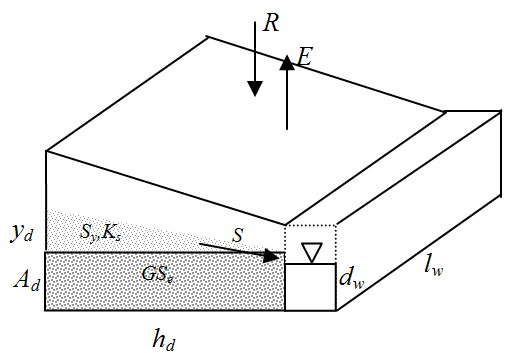# Groundwater-Surface Water Interactions

In many watersheds, surface waters and groundwater are hydraulically connected. A stream can contribute to groundwater recharge (i.e., a losing stream) or can gain water from the aquifer (i.e., a gaining stream) depending on the level of groundwater in the aquifer. Groundwater levels respond to natural recharge from precipitation, but can also be influenced by irrigation in the watershed, where a portion of this water may recharge the aquifer rather than be taken up by the target crop.  Pumping, of course, can draw down groundwater levels.

Of the four options to simulate groundwater-surface water interactions, the following describes the option where WEAP models these interactions by using a groundwater "wedge" connected to the river.To simulate groundwater interactions with surface waters, a stylized representation of the system can be used. Groundwater can be represented as a wedge that is symmetrical about the surface water body, such as a river; recharge and extraction from one side of the wedge will therefore represent half the total rate.

Total groundwater storage is first estimated using the assumption that the groundwater table is in equilibrium with the river; equilibrium storage for one side of the wedge, GSe, can be given as,

where hd (m) represents the distance that extends in a direction horizontally and at a right angle to the stream, lw (m) is the wetted length of the aquifer in contact with the stream, Sy is the specific yield of the aquifer, and Ad is the aquifer depth at equilibrium. An estimate of the height above which the aquifer lies or is drawn below the equilibrium storage height is given by yd, so the initial storage GS(0) in the aquifer at t =0 is given as,

The vertical height of the aquifer above or below the equilibrium position is given as,

Vertical height below the equilibrium position can be limited to the Maximum Head Difference (if it is set).  Limiting the head difference for losing streams might be necessary in cases where the aquifer is drawn down far below the river, in order to prevent unrealistically large volumes of water from being lost from the river.

The more the water table rises relative to the stream channel, the greater the seepage becomes to the stream. The more the water table falls relative the stream channel, the greater the loss of water from the stream channel to the aquifer. Total seepage from both sides of the river () is defined by,

where Ks (m/time) is an estimate of the saturated hydraulic conductivity of the aquifer and dw is an estimate of the wetted depth of the stream, which is time invariant. The wetted depth, together with the wetted length, approximate the area through which the seepage takes place. The saturated hydraulic conductivity controls the rate at which water moves toward or away from this seepage area. Once seepage is estimated, the groundwater storage at the end of the current time step is estimated as,

where E is the anthropogenic extraction from the aquifer that is associated with meeting water demand and R is recharge from precipitation.